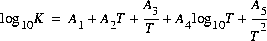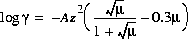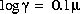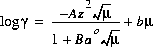[Next] [Previous] [Up] [Top]

Keywords

### SOLUTION_SPECIES

This keyword is used to define chemical reaction, log K, and activity-coefficient parameters for each aqueous species. Normally, this data block is included in the database file and only additions and modifications are included in the input file.

#### Example

```Line 0:  SOLUTION_SPECIES
Line 1a: SO4-2 = SO4-2
Line 2a:      log_k     0.0
Line 5a:      -gamma    5.0     -0.04
Line 1b: SO4-2 + 9H+ + 8e- = HS- + 4H2O
Line 2b:      log_k     33.652
Line 3b:      delta_h   -40.14
Line 5b:      -gamma    3.5     0.0
Line 1c: H2O = OH- + H+
Line 2c:      log_k     -14.000
Line 3c:      delta_h   13.362  kcal
Line 4c:      -a_e -283.971 -0.05069842 13323.0  102.24447 -1119669.0
Line 5c:      -gamma    3.5     0.0
Line 1d: HS-  = S2-2 + H+
Line 2d:      log_k     -14.528
Line 3d:      delta_h   11.4
Line 6:       -no_check
Line 7d:      -mole_balance    S(-2)2
```

#### Explanation

Line 0: SOLUTION_SPECIES

Keyword for the data block. No other data are input on the keyword line.

Line 1: Association reaction

Association reaction for aqueous species. The defined species must be the first species to the right of the equal sign. The association reaction must precede any identifiers related to the aqueous species. Reaction is identity reaction for primary master species.

Line 2: log_k log K

log_k--Identifier for log K at 25oC. Optionally, -log_k, logk, -l[og_k], or -l[ogk].

log K--Log K at 25oC for the reaction. Default 0.0. Log K must be 0.0 for primary master species.

Line 3: delta_h enthalpy, units

delta_h--Identifier for enthalpy of reaction at 25oC. Optionally, -delta_h, deltah, -d[elta_h], or -d[eltah].

enthalpy--enthalpy of reaction at 25oC for the reaction. Default 0.0.

units--Default units are kilojoules per mole. Units may be calories, kilocalories, joules, or kilojoules per mole. Only the energy unit is needed (per mole is assumed) and abbreviations of these units are acceptable. Explicit definition of units for all enthalpy values is recommended. The enthalpy of reaction is used in the van't Hoff equation to determine the temperature dependence of the equilibrium constant. Internally, all enthalpy calculations are performed with the units of kilojoules per mole.

Line 4: -analytical_expression A1, A2, A3, A4, A5

-analytical_expression--Identifier for coefficients for an analytical expression for the temperature dependence of log K. Optionally, analytical_expression, a_e, ae, -a[nalytical_expression], -a[_e], -a[e].

A1, A2, A3, A4, A5--Five values defining log K as a function of temperature in the expression, where T is in Kelvin.

Line 5: -gamma Debye-Hückel a, Debye-Hückel b

-gamma--indicates activity-coefficient parameters are to be entered. Optionally, -g[amma]. If -gamma is not input for a species, for charged species the Davies equation is used to calculate the activity coefficient:; for uncharged species the following equation is used. If -gamma is entered, then the equation from WATEQ (Truesdell and Jones, 1974) is used,. In these equations, g is the activity coefficient, m is ionic strength, and A and B are constants at a given temperature.

Debye-Hückel a--parameter ao in the WATEQ activity-coefficient equation.

Debye-Hückel b--parameter b in the WATEQ activity-coefficient equation.

Line 6: -no_check

-no_check--indicates the reaction equation defining aqueous species should not be checked for charge and elemental balance. Optionally, no_check, or -n[o_check]. By default, all equations are checked. The only exceptions might be polysulfide species which assume equilibrium with a solid phase; this assumption has the effect of removing solid sulfur from the mass-action equation. However, the identifier -mole_balance is needed to ensure that the proper number of atoms of each element are included in mole-balance equations (see -mole_balance).

Line 7: -mole_balance formula

-mole_balance--indicates the stoichiometry of the species will be defined explicitly. Optionally, mole_balance, mass_balance, mb, -m[ole_balance], -mass_balance, -m[b].

formula--chemical formula defining the stoichiometry of the species. Normally, both the stoichiometry and mass-action expression for the species are determined from the chemical equation that defines the species. Rarely, it may be necessary to define the stoichiometry of the species separately from the mass-action equation. The polysulfide species provide an example. These species are traditionally assumed to be in equilibrium with native sulfur. The activity of a pure solid is 1.0 and thus the term for native sulfur does not appear in the mass-action expression (Line 1d). The S2- species contains two atoms of sulfur, but the chemical equation indicates it is formed from species containing a total of one sulfur atom. The -mole_balance identifier is needed to give the correct stoichiometry. Note that unlike all other chemical formulas used in PHREEQC, the valence state of the element can and should be included in the formula (Line 7d). The example indicates that the polysulfide species will be summed into the S(-2) mole-balance equation in any initial solution calculations.

#### Notes

Line 1 must be entered first in the definition of a species. Additional sets of lines (lines 1-8 as needed) may be added to define all of the aqueous species. A log K must be defined for each species with either log_k (line 2) or -analytical_expression (line 4); default is 0.0, but is not meaningful except for primary master species. In this example, the following types of aqueous species are defined: (a) a primary master species, SO4-2, the reaction is an identity reaction and log K is 0.0; (b) a secondary master species, HS-, the reaction contains electrons; (c) an aqueous species that is not a master species, OH-; and (d) an aqueous species for which the chemical equation does not balance, S2-2.

By default, equation checking for charge and elemental balance is in force for each equation that is processed. Checking can only be disabled by using -no_check for each equation that is to be excluded from the checking process.

#### Example problems

The keyword SOLUTION_SPECIES is used in example problem 1. See also the listing of the default database file in Attachment B.

#### Related keywords

SOLUTION_MASTER_SPECIES and SOLUTION.

Example
Explanation
Notes
Example problems
Related keywords

User's Guide to PHREEQC - 07 MAY 96
[Next] [Previous] [Up] [Top]